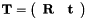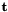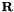OpenGV A library for solving calibrated central and non-central geometric vision problemsopengv::math Namespace Reference

The namespace of the math tools. More...

class  Bracket

class  Sturm

## Functions

rotation_t arun (const Eigen::MatrixXd &Hcross)
Arun's method for computing the rotation between two point sets. Core function . More...

transformation_t arun_complete (const points_t &p1, const points_t &p2)
Arun's method for complete point cloud alignment . The method actually does the same than threept_arun, but has a different interface. More...

rotation_t cayley2rot (const cayley_t &cayley)
Compute a rotation matrix from Cayley-parameters, following . More...

rotation_t cayley2rot_reduced (const cayley_t &cayley)
Compute a fake rotation matrix from Cayley-parameters, following . The rotation matrix is missing the scaling parameter of the Cayley-transform. This short form is useful for the Jacobian-based iterative rotation optimization of the eigensolver . More...

cayley_t rot2cayley (const rotation_t &R)
Compute the Cayley-parameters of a rotation matrix, following . More...

void gauss_jordan (std::vector< std::vector< double > * > &matrix, int exitCondition=0)
Sparse, fast Gauss Jordan elimination on matrices with a left square invertible block. More...

rotation_t quaternion2rot (const quaternion_t &quaternion)
Compute a rotation matrix from quaternion-parameters. Assumes that the quaternion has unit norm. More...

quaternion_t rot2quaternion (const rotation_t &R)
Compute the quaternion-parameters of a rotation matrix. More...

std::vector< double > o3_roots (const std::vector< double > &p)
The roots of a third-order polynomial. More...

std::vector< double > o4_roots (const Eigen::MatrixXd &p)
Ferrari's method for computing the roots of a fourth order polynomial. More...

std::vector< double > o4_roots (const std::vector< double > &p)
Ferrari's method for computing the roots of a fourth order polynomial. With a different interface. More...

## Detailed Description

The namespace of the math tools.

## Function Documentation

 rotation_t opengv::math::arun ( const Eigen::MatrixXd & Hcross )

Arun's method for computing the rotation between two point sets. Core function .

Parameters
 [in] Hcross The summation over the exterior products between the normalized points.
Returns
The rotation matrix that aligns the points.
 transformation_t opengv::math::arun_complete ( const points_t & p1, const points_t & p2 )

Arun's method for complete point cloud alignment . The method actually does the same than threept_arun, but has a different interface.

Parameters
 [in] p1 The points expressed in the first frame. [in] p2 The points expressed in the second frame.
Returns
The Transformation from frame 2 to frame 1 (, withbeing the position of frame 2 seen from frame 1, andbeing the rotation from frame 2 to frame 1).
 rotation_t opengv::math::cayley2rot ( const cayley_t & cayley )

Compute a rotation matrix from Cayley-parameters, following .

Parameters
 [in] cayley The Cayley-parameters of a rotation.
Returns
The 3x3 rotation matrix.
 rotation_t opengv::math::cayley2rot_reduced ( const cayley_t & cayley )

Compute a fake rotation matrix from Cayley-parameters, following . The rotation matrix is missing the scaling parameter of the Cayley-transform. This short form is useful for the Jacobian-based iterative rotation optimization of the eigensolver .

Parameters
 [in] cayley The Cayley-parameters of the rotation.
Returns
The false 3x3 rotation matrix.
 void opengv::math::gauss_jordan ( std::vector< std::vector< double > * > & matrix, int exitCondition = 0 )

Sparse, fast Gauss Jordan elimination on matrices with a left square invertible block.

Parameters
 [in] matrix The matrix. [in] exitCondition The last row we process when stepping up.
 std::vector opengv::math::o3_roots ( const std::vector< double > & p )

The roots of a third-order polynomial.

Parameters
 [in] p The polynomial coefficients (poly = p*x^3 + p*x^2 ...).
Returns
The roots of the polynomial (only real ones).
 std::vector opengv::math::o4_roots ( const Eigen::MatrixXd & p )

Ferrari's method for computing the roots of a fourth order polynomial.

Parameters
 [in] p The polynomial coefficients (poly = p(0,0)*x^4 + p(1,0)*x^3 ...).
Returns
The roots of the polynomial (only real ones).
 std::vector opengv::math::o4_roots ( const std::vector< double > & p )

Ferrari's method for computing the roots of a fourth order polynomial. With a different interface.

Parameters
 [in] p The polynomial coefficients (poly = p*x^4 + p*x^3 ...).
Returns
The roots of the polynomial (only real ones).
 rotation_t opengv::math::quaternion2rot ( const quaternion_t & quaternion )

Compute a rotation matrix from quaternion-parameters. Assumes that the quaternion has unit norm.

Parameters
 [in] quaternion The quaternion-parameters of a rotation.
Returns
The 3x3 rotation matrix.
 cayley_t opengv::math::rot2cayley ( const rotation_t & R )

Compute the Cayley-parameters of a rotation matrix, following .

Parameters
 [in] R The 3x3 rotation matrix.
Returns
The Cayley-parameters.
 quaternion_t opengv::math::rot2quaternion ( const rotation_t & R )

Compute the quaternion-parameters of a rotation matrix.

Parameters
 [in] R The 3x3 rotation matrix.
Returns
The quaternion-parameters.